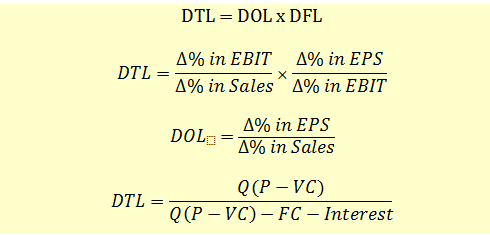# Degree of Total Leverage

Using the formulas for Degree of Operating Leverage (DOL) and Degree of Financial Leverage (DFL), we can calculate the degree of total leverage (DTL) for the firm as follows:In the above formula, you can observe that if there are no fixed operating costs and fixed financing costs, there is no leverage, and DTL will be equal to 1.